# Orlicz space

(diff) ← Older revision | Latest revision (diff) | Newer revision → (diff)

A Banach space of measurable functions introduced by W. Orlicz . Let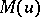and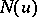be a pair of complementary-functions (see Orlicz class) and letbe a bounded closed set in. The Orlicz space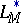is the set of Lebesgue-measurable functionsonfor whichAn Orlicz space is a complete normed space with respect to the norm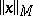, which is called the Orlicz norm. When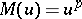,,coincides with the Riesz spaceand, up to a scalar factor,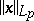coincides with.

If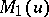and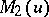are-functions, then the inclusion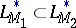holds if and only if, for a certainand all sufficiently large, the inequality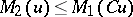is fulfilled. For every Orlicz spacethe inclusionshold. Every summable function belongs to some Orlicz space.

The spaceis separable if and only ifsatisfies the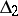-condition (cf. Orlicz class). In general,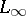is not dense in, and the closure ofinis denoted by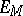and is always separable. If, then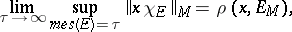whereIfandare complementary-functions and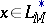,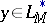, then the following analogue of the Hölder inequality holds: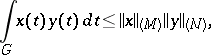where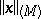is the Luxemburg norm. Every continuous linear functionaloncan be represented in the form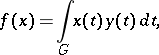where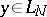and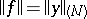.

The compactness criteria of M. Riesz and A.N. Kolmogorov for the spaces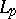can also be applied to. The following conditions are equivalent:

1) the spaceis reflexive;

2)andsatisfy the-condition;

3) an unconditional basis exists in;

4) the Haar system forms an unconditional basis in;

5) the trigonometric system is a basis inand the Haar system is a basis in.

A sequence spaceis defined in the same way, but the properties ofdepend on the asymptotics of the functionat 0. Many of the geometric properties ofand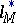are studied in ; for example, for any function, the set of allsuch that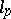is isomorphically imbeddable incan be found.

Orlicz spaces are used in the study of the properties of integral operators, the theory of differentiable functions of several variables and in other areas of analysis.

How to Cite This Entry:
Orlicz space. Encyclopedia of Mathematics. URL: http://encyclopediaofmath.org/index.php?title=Orlicz_space&oldid=17861
This article was adapted from an original article by E.M. Semenov (originator), which appeared in Encyclopedia of Mathematics - ISBN 1402006098. See original article